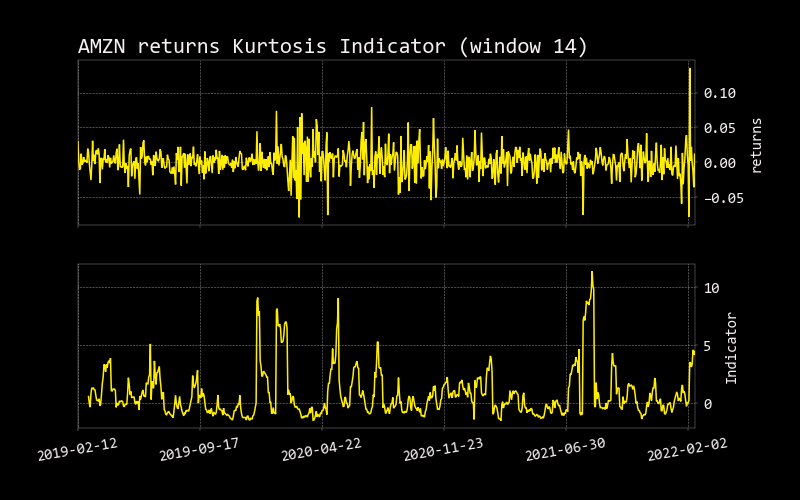# Kurtosis

``````usage: kurtosis [-l N_LENGTH] [--export {csv,json,xlsx}] [-h]
``````

Kurtosis is a measure of the “tailedness” of the probability distribution of a real-valued random variable. Like skewness, kurtosis describes the shape of a probability distribution and there are different ways of quantifying it for a theoretical distribution and corresponding ways of estimating it from a sample from a population. Different measures of kurtosis may have different interpretations.

For research on this topic, visit: https://www.styleadvisor.com/resources/statfacts/kurtosis

Kurtosis identifies where the volatility risk came from in a distribution of returns. Kurtosis improves one’s understanding of volatility risk.

Kurtosis is also known as the fourth moment of the distribution, used in conjunction with mean, standard deviation, and skewness to understand the shape of a distribution of returns. In its base case, kurtosis has a neutral value of 3.0. The calculation is frequently modified by the second term in the equation below, which scales kurtosis so that the baseline, neutral value is 0.0.

``````optional arguments:
-l N_LENGTH, --length N_LENGTH
length (default: 14)
--export {csv,json,xlsx}
Export dataframe data to csv,json,xlsx file (default: )
-h, --help            show this help message (default: False)
``````